Next: Neural Gas plus Competitive Up: Soft Competitive Learning without Previous: Neural Gas

# Competitive Hebbian Learning

This method (Martinetz and Schulten, 1991; Martinetz, 1993) is usually not used on its own but in conjunction with other methods (see sections 5.3 and 5.4). It is, however, instructive to study competitive Hebbian learning on its own. The method does not change reference vectors at all (which could be interpreted as having a zero learning rate). It only generates a number of neighborhood edges between the units of the network. It was proved by Martinetz (1993) that the so generated graph is optimally topology-preserving in a very general sense. In particular each edge of this graph belongs to the Delaunay triangulation corresponding to the given set of reference vectors. The complete competitive Hebbian learning algorithm is the following:

1.
Initialize the setto contain N unitswith reference vectors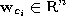chosen randomly according to.

Initialize the connection set,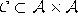, to the empty set: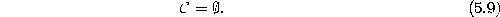2.
Generate at random an input signalaccording to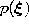.
3.
Determine units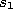andsuch that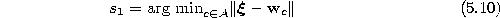and4.
If a connection betweenand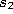does not exist already, create it:5.
Continue with step 2 unless the maximum number of signals is reached.

Figure 5.3 shows some stages of a simulation for a simple ring-shaped data distribution. Figure 5.4 displays the final results after 40000 adaptation steps for three other distribution.Figure 5.3:   Competitive Hebbian learning simulation sequence for a ring-shaped uniform probability distribution. a) Initial state. b-f) Intermediate states. g) Final state. h) Voronoi tessellation corresponding to the final state. Obviously, the method is sensitive to initialization since the initial positions are always equal to the final positions.Figure:   Competitive Hebbian learning simulation results after 40000 input signals for three different probability distributions (described in the caption of figure 4.4).Next: Neural Gas plus Competitive Up: Soft Competitive Learning without Previous: Neural Gas

Bernd Fritzke
Sat Apr 5 18:17:58 MET DST 1997